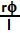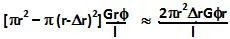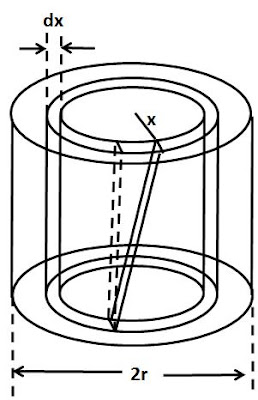## Tuesday, April 14, 2009

### Irodov Problem 1.305

a)The shearing moment acting on the cylinder, causes shear stress (check the definition of shear modulus here) resulting in the cylinder to twist and thus in a shear strain. As the cylinder twists
an angleresulting in the upper surface to shift a distance ofrelative to the lower surface as shown in the figure. Thus, the shear strain is given by. This, shear strain will result in a shear stress acting tangential to the surface given by. The net tangential force acting on the cylinder is given by the stress times the area of cross section given by,Since this force acts at a distance r from the axis, the twisting moment is given by,b)For this part consider an infinitesimally thin vertical section of the solid cylinder at a distance x from the center and of thickness dx. As solved in part a of the problem, the twisting moment acting on this section will be given by,